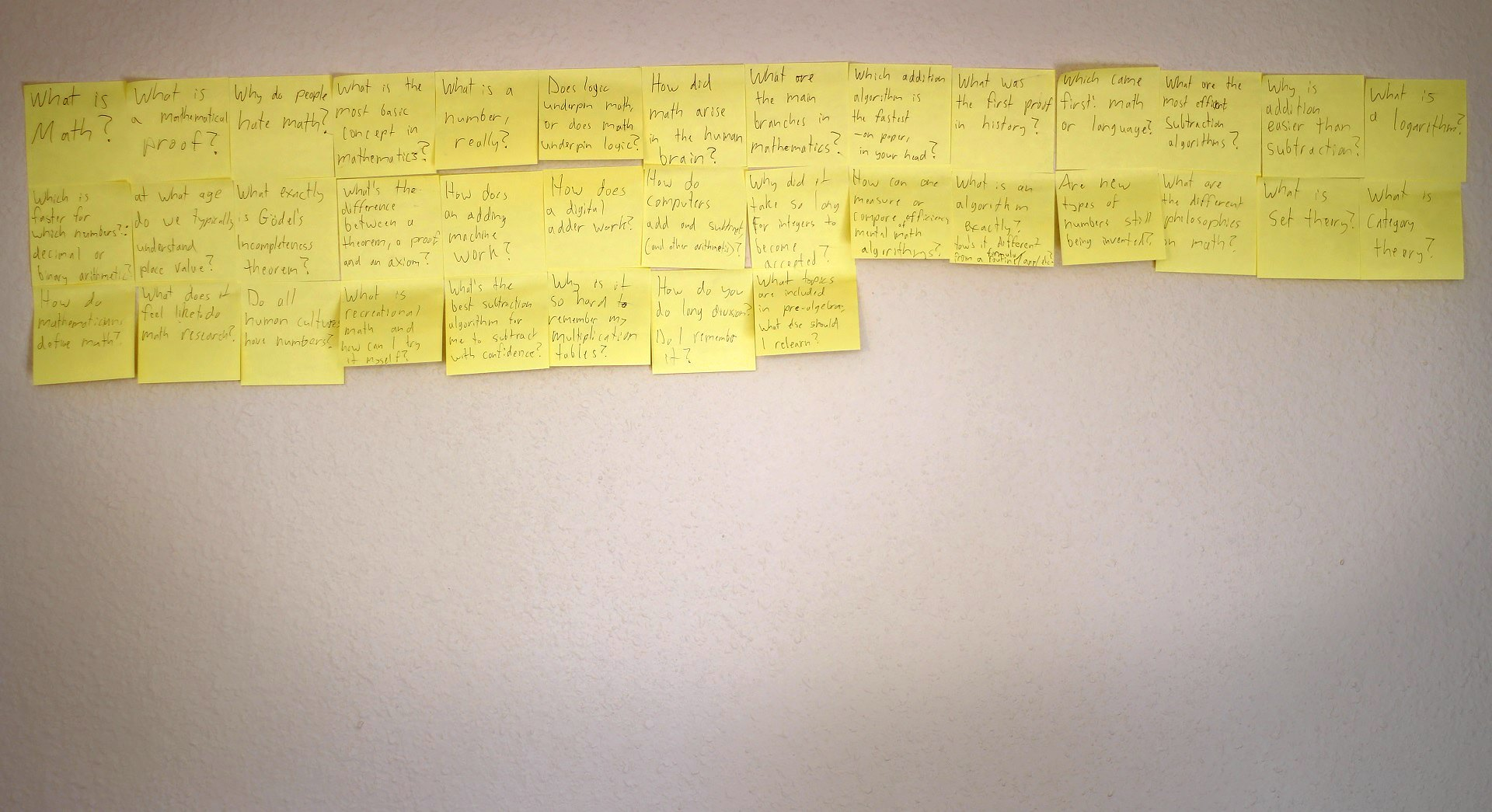# Facing a wall of questions (Math Immersion Month Day 7)

Today I gathered up my math questions and stuck them on my living room wall – now dubbed the Wall of Questions! I wrote a bit about the idea on my blog post for day 1.

## Wall of Questions

I’m not worried about answering these questions just yet. (Besides, I’ve already half-answered a few of them). The point is just to keep track of them (and decorate the walls of my livingroom). Questions written down so far:• What is math?
• What is a mathematical proof?
• Why do people hate math?
• What is the most basic concept in mathematics?
• What is a number, really?
• Does logic underpin math or does math underpin logic?
• How did math arise in the human brain?
• What are the main branches in mathematics?
• What was the first proof in history?
• Which came first: math or language?
• What are the most efficient subtraction algorithms?
• Why is addition easier than subtraction?
• What is a logarithm?
• Which is faster: decimal or binary arithmetic?
• At what age do we typically understand place value?
• What exactly is Godel’s incompleteness theorem?
• What’s the difference between a theorem, a proof and an axiom?
• How does an adding machine work?
• How does a digital adder work?
• What topics are included in pre-algebra; what else should I relearn?
• Why did it take so long for integers to become accepted?
• How can you measure or compare the efficiency of mental math algorithms? What is an algorithm exactly? How’s it different from a formula or a routine?
• Are new types of new types of numbers still being invented?
• What are the different philosophies in math?
• What is set theory?
• What is category theory?
• How do mathematicians define math?
• What does it feel like to do math research?
• Do all human cultures have numbers?
• What is recreational math and how can I try it/enjoy it?
• Why is it so hard to remember my multiplication tables?
• How do you do long division? Do I remember it?
• How do computers add and subtract (and do other calculations)?

## Time breakdown

• Video production time: 1 hour 27 min
• Script writing/preparation: 3 min
• Filming/setup: 24 min
• Video editing: 45 min
• Publishing: 15 min
• Math study time: 39 min
• Practice problems: none
• General research: 39 min

## Credits### Written by Liz Krane

Liz is a full-time nerd, sharing everything she learns as she tries to learn everything!

Updated